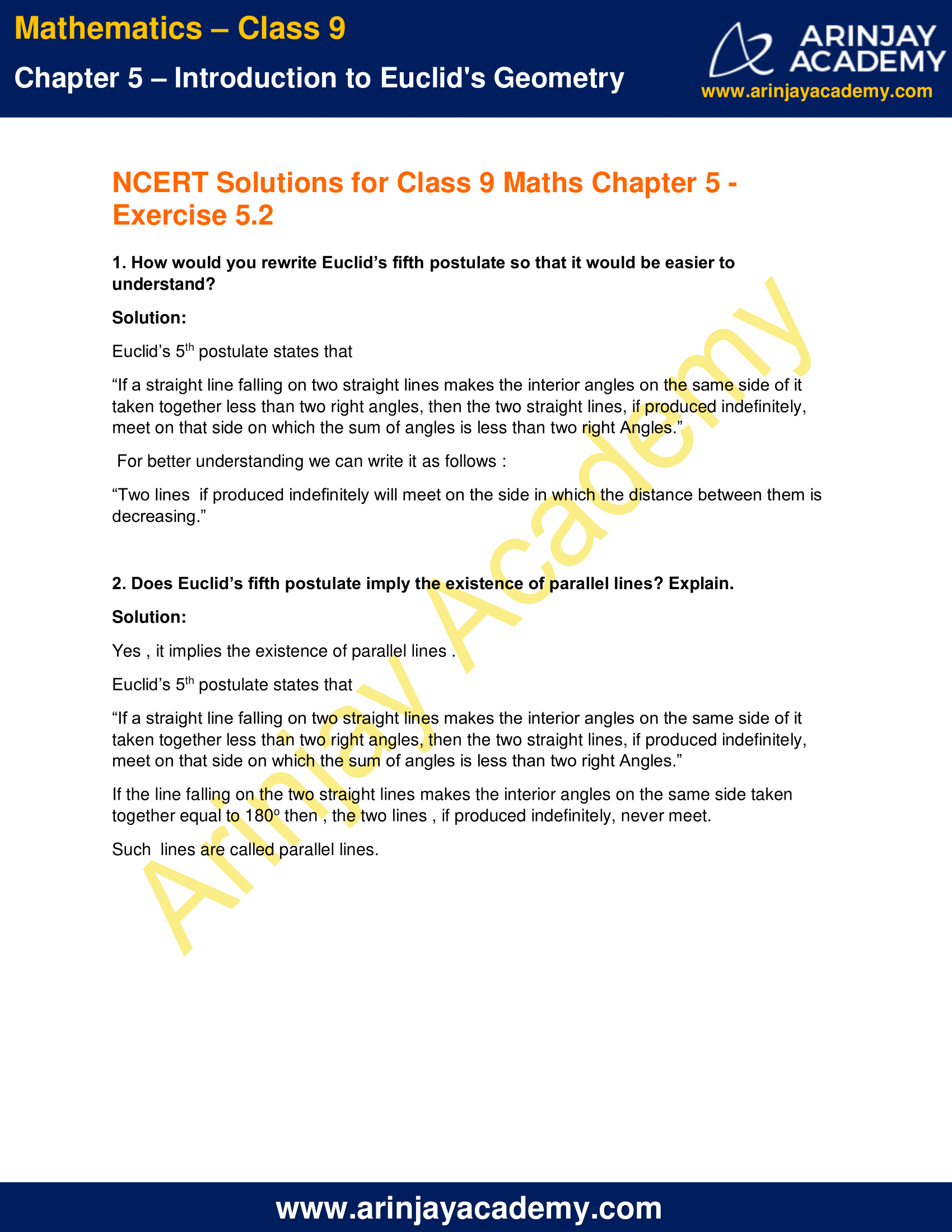# NCERT Solutions for Class 9 Maths Chapter 5 Exercise 5.2

Download NCERT Solutions for Class 9 Maths Chapter 5 Exercise 5.2 – Introduction to Euclid’s Geometry. This Exercise contains 2 questions, for which detailed answers have been provided in this note. In case you are looking at studying the remaining Exercise for Class 9 for Maths NCERT solutions for Chapter 6 or other Chapters, you can click the link at the end of this Note.

### NCERT Solutions for Class 9 Maths Chapter 5 Exercise 5.2NCERT Solutions for Class 9 Maths Chapter 5 Exercise 5.2

1. How would you rewrite Euclid’s fifth postulate so that it would be easier to understand?

Solution:

Euclid’s 5th postulate states that
“If a straight line falling on two straight lines makes the interior angles on the same side of it taken together less than two right angles, then the two straight lines, if produced indefinitely, meet on that side on which the sum of angles is less than two right Angles.”
For better understanding we can write it as follows :
“Two lines  if produced indefinitely will meet on the side in which the distance between them is decreasing.”

2. Does Euclid’s fifth postulate imply the existence of parallel lines? Explain.

Solution:

Yes , it implies the existence of parallel lines .
Euclid’s 5th postulate states that
“If a straight line falling on two straight lines makes the interior angles on the same side of it taken together less than two right angles, then the two straight lines, if produced indefinitely, meet on that side on which the sum of angles is less than two right Angles.”
If the line falling on the two straight lines makes the interior angles on the same side taken together equal to 180o then , the two lines , if produced indefinitely, never meet.
Such  lines are called parallel lines.

NCERT Solutions for Class 9 Maths Chapter 5 Exercise 5.2 – Introduction to Euclid’s Geometry, has been designed by the NCERT to test the knowledge of the student on the topic – Equivalent Versions of Euclid’s Fifth Postulate

The next Exercise for NCERT Solutions for Class 9 Maths Chapter 6 Exercise 6.1 – Lines and Angles can be accessed by clicking here.

Download NCERT Solutions for Class 9 Maths Chapter 5 Exercise 5.2 – Introduction to Euclid’s Geometry